# Electronics and Communication Engineering - Digital Electronics

26.

9's complement of 1210 is

 A. 2110 B. 8710 C. 7810 D. 7610

Answer: Option B

Explanation:

99 - 12 = 87.

27.

The minterm designation for ABCD is

 A. m8 B. m10 C. m14 D. m15

Answer: Option D

Explanation:

ABCD = 1111 = m15 .

28.

Read the following statements

1. The circuitry of ripple counter is more complex than that of synchronous counter.
2. The maximum frequency of operation of ripple counter depends on the modulus of the counter.
3. The maximum frequency of operation of synchronous counter does not depend on the modulus of the counter.
Which of the above statements are correct?

 A. 1 only B. 1, 2 C. 1, 2, 3 D. 2, 3

Answer: Option D

Explanation:

Circuitry of ripple counter is simpler than that of synchronous counter.

29.

The minterm designation for ABCD is

 A. m11 B. m13 C. m15 D. m16

Answer: Option C

Explanation:

ABCD = 1111 = m15 .

30.

The logic circuit of the given figure is equivalent to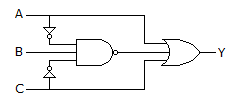A.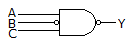B.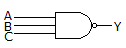C.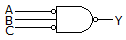D.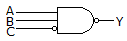Answer: Option C

Explanation: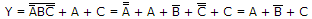In the given figure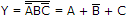.

#### Current Affairs 2021

Interview Questions and Answers